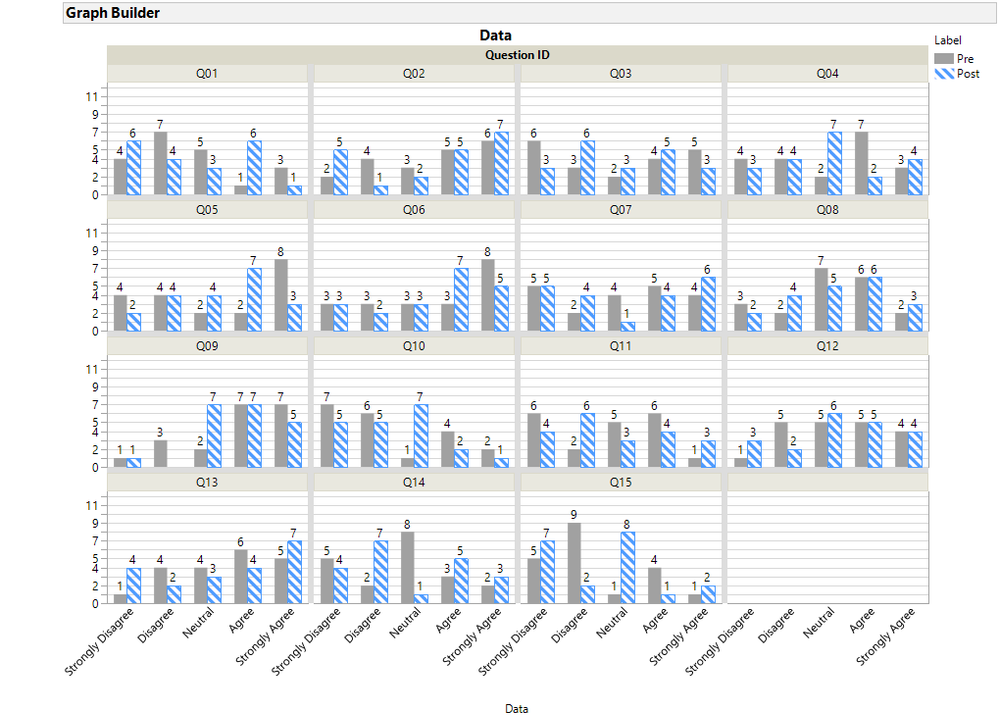Choose Language Hide Translation Bar
Highlighted

## Pre/Post-Survey Bar Graphs in JMP

All,

I am looking for a way to show pre- and post- survey questions next to each other in a bar graph. The possible responses (strongly disagree throuhg strongly agree) should be in the x-axis, and the number of recipients responding in each of those catagories should be in y-axis. However, I want to show the values of each response value next to each other to show the before and after affect of the exercise. I can get the groups next to each other with graph builder, but I can't get the "strongly disagree" values to be grouped together, etc. Any help would be greatly appreciated.

- Nathan

2 REPLIES 2
Highlighted

## Re: Pre/Post-Survey Bar Graphs in JMP

This would be easier to answer is there were more details, for example what do you mean by groups? Groups of questions or groups of respondents.  Often it is easier to arrange bars when the data is stacked.

If you can show the layout of your data without showing the specific values that would be helpful.

Highlighted

## Re: Pre/Post-Survey Bar Graphs in JMP

Below is a script that generates a table simulating data for 15 questions, three for each of 5 topics and 20 respondents. I have no idea how your data is organized. Thsi table has 300 rows (20 x 5 x  3), identifier columns (Respondent, Question ID, Gender, Topic, Pre and Post).

So that the pre/post bars can be side-by-side, the data needs to be stacked, in other words, Pre/Post is a label for each response.

Below is one display/example display. The script to generate some data, stack the data, then create this graph is below the picture.``````Names Default to Here(1);

//Simulate a table of survey responses 20 respondents 15 questions: 3 questions per topic and 5 topics
//Gender is a respondent group, Topic is a question group
//responses are the Likert Scale
dt = New Table("Survey",
New Column("Respondent", Character, Nominal),
New Column ("Gender", Character, Nominal),
New Column("Question ID", Character, Ordinal),
New Column("Topic", Character, Nominal),
New Column("Pre", Ordinal),
New Column("Post", Ordinal)
);

dt:Pre << Value Labels(
{1 = "Strongly Disagree", 2 = "Disagree", 3 = "Neutral", 4 = "Agree",
5 = "Strongly Agree"}
);

dt:Post << Value Labels(
{1 = "Strongly Disagree", 2 = "Disagree", 3 = "Neutral", 4 = "Agree",
5 = "Strongly Agree"}
);

dt:Respondent << Set Each Value( Right("00" || Char(Sequence(1,20,1,15)),3) );
dt:Question ID  << Set Each Value("Q" || Right("0"||char(Sequence(1,15,1,1)),2));
dt:Gender[1::150]="F";
dt:Gender[151::300]="M";
tlist = {"Food", "Technology", "Environment", "Energy", "Education"  };
dt:Topic << Set Each Value( tlist[Sequence(1,5,1,3)]);
dt:Pre   << Set Each Value(Random Integer(1,5));
//create some interest
dt:Post  << Set Each Value(If(:Gender=="F" & :Pre==5, 5,
:Gender=="F", :Pre + 1,
:Gender=="M" & :Pre==1, 1,
:Gender=="M", :Pre -1
));

dt:Topic << Set Property("Value Ordering",
{"Food", "Technology", "Environment", "Energy", "Education"}
);

//Step2: Stack the table - the label for the data will be Pre and Post and as an Overlay variable for
//GraphBuilder the bars will be side by side
dt_stck= dt << Stack(
columns( :Pre, :Post ),
Source Label Column( "Label" ),
Stacked Data Column( "Data" ), Output Table Name("Stacked Pre Post Survey")
);

//Make sure Pre is ordered before Post
dt_stck:Label << Set Property("Value Ordering", {"Pre", "Post"});

gb = dt_stck << Graph Builder(      // draw graph
Size( 881, 707 ),
Show Control Panel( 0 ),
Graph Spacing( 6 ),
Variables( X( :Data ), Wrap( :Question ID ), Overlay( :Label ) ),
Elements(
Bar( X, Legend( 13 ), Summary Statistic( "N" ), Label( "Label by Value" ) )
),
SendToReport(
Dispatch(
{},
"",
ScaleBox,
{Min( 0 ), Max( 12.5 ), Inc( 1 ), Minor Ticks( 0 ),
Label Row( Show Major Grid( 1 ) )}
),
Dispatch(
{},
"400",
ScaleBox,
{Legend Model(
13,
Properties( 0, {Fill Color( -10592673 )}, Item ID( "Pre", 1 ) ),
Properties(
1,
{Fill Color( 37 ), Fill Pattern( "left slant heavy b" )},
Item ID( "Post", 1 )
)
)}
)
)
);
``````

Article Labels

There are no labels assigned to this post.